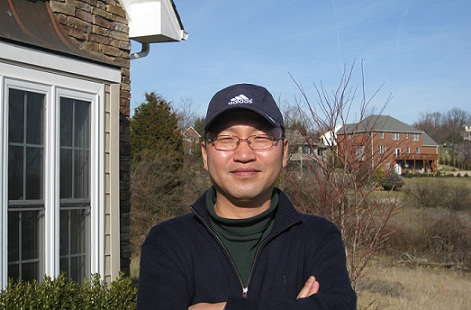### Research Interests:

• Fully Nonlinear Partial Differential Equations

• Degenerate Partial Differential Equations(Porous medium equation, Monge Ampére Equations)

• Curvature flow (Gauss Curvature Flows, Scalar Curvature Flows)

• Free Boundary Problems (Obstacle Problems, Flame Propagation)

• Homogenization, Random Media

• Integro-Differential Equations, Nonlocal Equations

### Lectures (VOD):

• Korea PDE Winter School , 2011

Regularity Theory on the Partial Differential Equations: Schauder theory for C^{2,α}-estimate, De Giorgi Theory for Harnack and Hölder Estimate for PDE of Divergence type, Krylov-Safanov for Harnack and Hölder Estimate for PDE of Non Divergence type